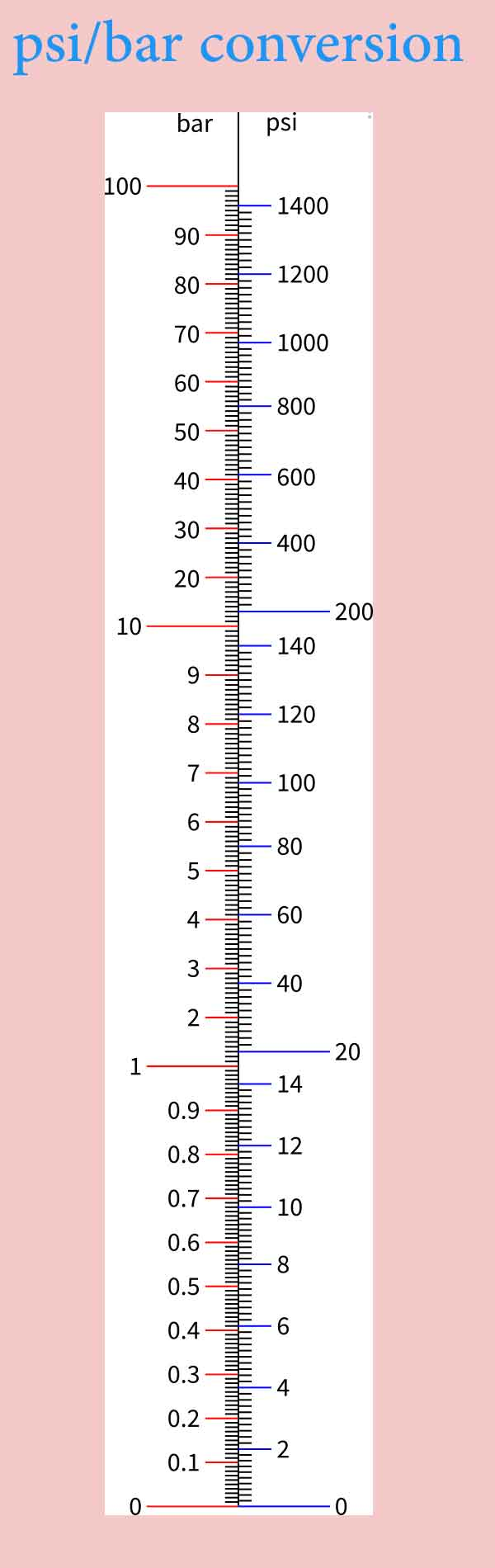# Psi to Bar Unit Converter - 1 Psi in Bar

This calculator allows you to convert from Psi to Bar and in a reverse direction. To convert from Psi to Bar, enter the amount of Psi into the first input and to convert from Bar to Psi, enter the amount of Bar into the second input.

1 Psi = 0.068947572931783 Bar

## Psi to Bar?

### How to convert from Psi to Bar?

To convert Psi to Bar: Every 1 Psi equals 0.068947572931783 Bar. For example, 100 Psi equal 100 * 0.068947572931783 = 6.8947572931783 Bar and so on..

Psi to Pascal | Psi to Pound-force/square inch | Psi to Standard atmosphere | Psi to Atmosphere technical | Psi to Torr |

## What is PSI?

PSI is Pound-force per Square Inch (lbf/in2). It is a unit of pressure used in England and USA.

It is the primary unit for measuring pressure in USA. Also, it is widely used in the UK.

1 PSI is equal to 0.0689476 bar. So to convert from PSI to bar multiply by this number. For example, 1000 PSI = 1000 x 0.0689476= 68.9476 bars.

## Bar

What is Bar?

Bar is a unit of pressure in the metric system but not in the SI system. It is equal to 100,000 Pa and is slightly less than the atmospheric pressure on earth at the sea level.

The bar is equal to 14.5038 PSI and to convert from bar to PSI multiply by this number.

## psi to bar conversion scale## Psi to Bar Conversions Table,